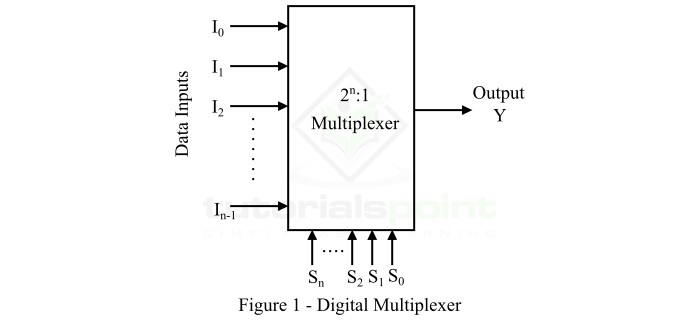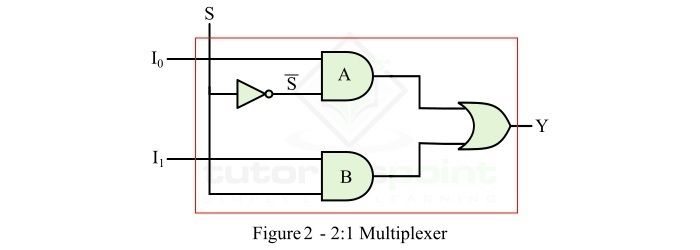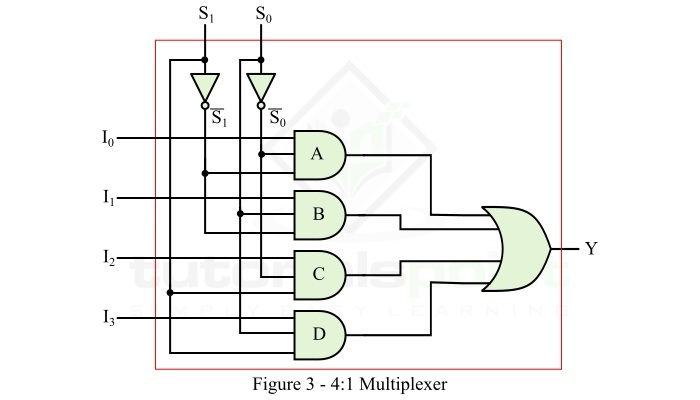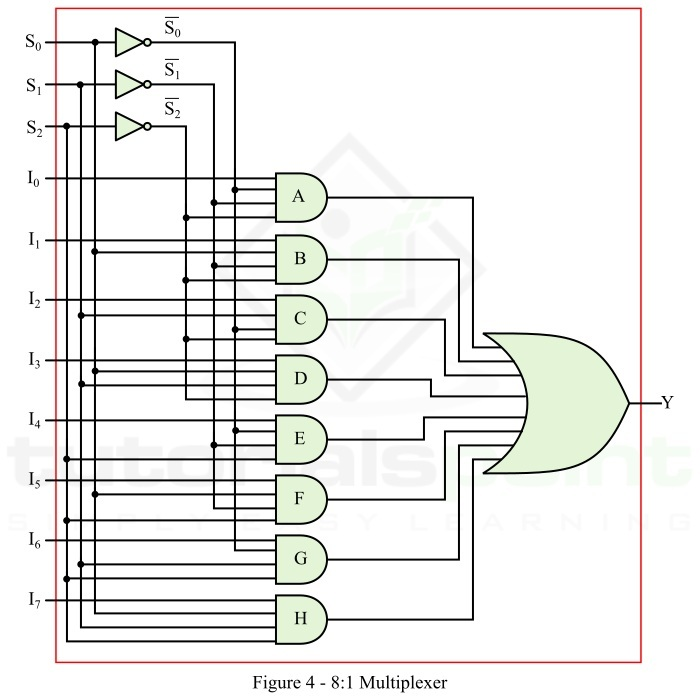# Multiplexer Design Procedure and Applications

A multiplexer (MUX) is a digital combinational logic circuit that accepts multiple data inputs and allows only one of them at a time to transmit over an output channel. A multiplexer consists of 2n input lines, n select lines, and one output line. In a multiplexer, the routing of the desired data input to the output channel is done by the logic level applied to the select lines. The functional block diagram of a multiplexer is shown in Figure-1.This article is primarily meant for explaining the design procedure of different types of multiplexer circuits. More specifically, here we will discuss the design procedure of the following three types of multiplexer −

• 2:1 Multiplexer
• 4:1 Multiplexer
• 8:1 Multiplexer

So let us now discuss the designing of each of these three types of multiplexer.

## Designing a 2:1 Multiplexer

A 2:1 multiplexer (MUX) is one which has 2 input lines (I0 and I1), 1 select line (S), and 1 output line (Y). The logic level applied to the select line S determines which data input will pass through the output line.

For determining the Boolean expression for output (Y) of the 2:1 multiplexer and its logic circuit implementation, we first need its function table (truth table) that gives information about operation of the circuit. The function table of the 2:1 multiplexer with data input I0 and I1 is shown below.

Select Line (S) Output (Y)
0 I0
1 I1

Using this truth table, we can write the logic expression for the output of 2:1 MUX as,

$$Y=\bar{S}I_{0}+SI_{1}$$

To implement this logic expression, we require two AND gates, one NOT gate, and one OR gate. The logic circuit of the 2:1 MUX is shown in Figure-2.### Operation

This logic circuit of the 2:1 MUX shown in figure-2 will work as follows −

• When S = 0, the AND gate A is enabled and the AND gate B is disabled. Therefore, the output Y = I0.

• When S = 1, the AND gate A is disabled and the AND gate B is enabled. Therefore, the output Y = I1.

This is how, we can design a 2:1 multiplexer.

## Designing a 4:1 Multiplexer

A 4:1 multiplexer (MUX) is the MUX which has 4 input lines (I0, I1, I2, and I3), 2 select line (S0 and S1), and 1 output line (Y). The logic levels applied to the select lines S0 and S1 determine which data input will transmit to the output line.

For determining the Boolean expression for output (Y) of the 4:1 multiplexer and its logic circuitry, we require its function table (truth table) that provides information about the operation of its circuit. The function table of the 4:1 multiplexer with data inputs I0, I1, I2, and I3 is given below.

Select Lines Output(Y)
S1 S0
0 0 I0
0 0 I1
1 0 I2
1 1 I3

Using this truth table, we can write the logic expression for the output of 4:1 MUX as,

$$Y=\bar{S_{1}}\bar{S_{0}}I_{0}+\bar{S_{1}}S_{0}I_{1}+S_{1}\bar{S_{0}}I_{2}+S_{1}S_{0}I_{3}$$

To implement this logic expression, we require four AND gates, two NOT gates, and one OR gate. Thus, the logic circuit of the 4:1 MUX is shown in Figure-3.### Operation

This logic circuit of the 4:1 MUX shown in Figure-3 will work as follows −

• When S1 = 0 and S0 = 0, the AND gate A is enabled, and the AND gates B, C, and D are disabled. So, the output Y = I0.

• When S1 = 0 and S0 = 1, the AND gate B is enabled, and the AND gates A, C, and D are disabled. So, the output Y = I1.

• When S1 = 1 and S0 = 0, the AND gate C is enabled, and the AND gates A, B, and D are disabled. So, the output Y = I2.

• When S1 = 1 and S0 = 1, the AND gate D is enabled, and the AND gates A, B, and C are disabled. So, the output Y = I3.

In this way, we can design a 4:1 multiplexer.

## Design 8:1 Multiplexer

An 8:1 multiplexer (MUX) is a combination logic circuit which has 8 input lines (I0, I1, I2, I3, I4, I5, I6, and I7), 3 select line (S0, S1, and S2), and 1 output line (Y). The logic levels applied to the select lines S0, S1, and S2 determine which data input will transmit to the output line.

To determine the logic expression for output (Y) of the 8:1 multiplexer and its logic circuitry, we require its function table (truth table) which provides information about the operation of its logic circuit.

The function table of the 8:1 multiplexer with data inputs I0, I1, I2, I3, I4, I5, I6, and I7 and select lines, S0, S1, and S2 is given below.

Select Lines Output (Y)
S2 S1 S0
000I0
001I1
010I2
011I3
100I4
101I5
110I6
111I7

By using this function table, we can write the logic expression for the output of 8:1 MUX as,

$$Y=\bar{S_{2}}\bar{S_{1}}\bar{S_{0}}I_{0}+\bar{S_{2}}\bar{S_{1}}S_{0}I_{1}+\bar{S_{2}}S_{1}\bar{S_{0}}I_{2}+\bar{S_{2}}S_{1}S_{0}I_{3}+S_{2}\bar{S_{1}}\bar{S_{0}}I_{4}+S_{2}\bar{S_{1}}S_{0}I_{5}+S_{2}S_{1}\bar{S_{0}}I_{6}+S_{2}S_{1}S_{0}I_{7}$$

To implement this logic expression, we require eight AND gates, three NOT gates, and one OR gate. Therefore, the logic circuit of the 8:1 MUX is shown in Figure-4.### Operation

This logic circuit of the 8:1 MUX shown in Fgure-4 will operate as follows −

• When S2 = 0, S1 = 0, and S0 = 0, the AND gate A is enabled, and all the other AND gates in the logic circuit are disabled. So, the output Y = I0.

• When S2 = 0, S1 = 0, and S0 = 1, the AND gate B is enabled, and all the other AND gates in the logic circuit are disabled. So, the output Y = I1.

• When S2 = 0, S1 = 1, and S0 = 0, the AND gate C is enabled, and all the other AND gates in the logic circuit are disabled. So, the output Y = I2.

• When S2 = 0, S1 = 1, and S0 = 1, the AND gate D is enabled, and all the other AND gates in the logic circuit are disabled. So, the output Y = I3.

• When S2 = 1, S1 = 0, and S0 = 0, the AND gate E is enabled, and all the other AND gates in the logic circuit are disabled. So, the output Y = I4.

• When S2 = 1, S1 = 0, and S0 = 1, the AND gate F is enabled, and all the other AND gates in the logic circuit are disabled. So, the output Y = I5.

• When S2 = 1, S1 = 1, and S0 = 0, the AND gate G is enabled, and all the other AND gates in the logic circuit are disabled. So, the output Y = I6.

• When S2 = 1, S1 = 1, and S0 = 1, the AND gate H is enabled, and all the other AND gates in the logic circuit are disabled. So, the output Y = I7.

In this way, we can design an 8:1 multiplexer.

## Applications of Multiplexers

The multiplexer is one of the widely combinational logic circuits in digital systems. Some important applications of the multiplexers are listed below.

• Multiplexer is used as data selector device.

• Multiplexers are used in communication systems to increase the efficiency of the system.

• Multiplexers are used in telephone networks for integration of several audio signals on a single transmission line.

• To maintain large amount of data, multiplexers are also used in computer memory systems.

• Multiplexers are also employed in TV broadcasting systems.

• Multiplexers are used in satellite communication and GPS (Global Positioning System).

• Multiplexers are also used in PLC (Programmable Logic Control) systems, etc.

This is all about the multiplexer design procedure, and applications of multiplexers.

Updated on: 19-Apr-2023

3K+ Views